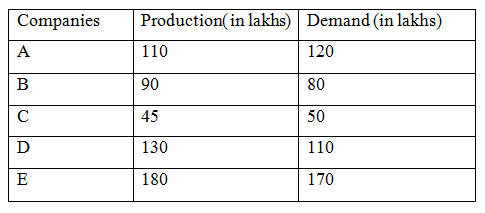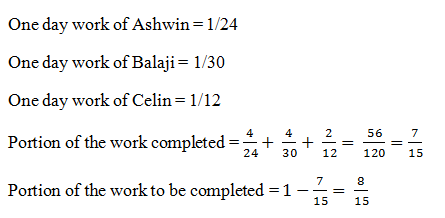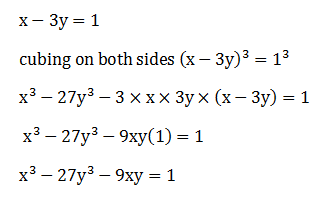# SSC CGL 2018 Practice Test Papers | Quantitative Aptitude (Day-54)

Dear Aspirants, Here we have given the Important SSC CGL Exam 2018 Practice Test Papers. Candidates those who are preparing for SSC CGL 2018 can practice these questions to get more confidence to Crack SSC CGL 2018 Examination.

[WpProQuiz 3628]

Click “Start Quiz” to attend these Questions and view Explanation

1) What is the value of √-36 ×√- 16 ?

a) 24

b) -24

c) ±24

d) Cannot be determined

2) A certain number of women can do a piece of work in 8 days. They start the work together but everyday 10 women left the work. The total work was completed in 12 days.Find the number of women started the work?

a) 165

b) 220

c) 320

d) 150

3) Ashwin can do a piece of work in 24 days. Balaji can do the same work in 30 days. Celin can do the same work in 12 days. Ashwin and Balaji started the work and worked for 4 days and they left. Then Celin alone do the work for 2 days. What is the total portion of the work to be completed?

a) 8/23

b) 8/15

c) 4/20

d) None of these

4)  Sharma has two sons. The age of Sharma is thrice of the sum of age of his two sons.  The product of the ages of sons is 24 and their age difference is 2 years. What is the age of Sharma five years hence?

a) 45

b) 40

c) 35

d) 20

5) A vendor sells goods at a loss of 5 %. If he sells it for Rs. 30 more, he will gain 10% of the cost price. What should be the Selling price if the seller wants to have 30 % profit?

a) 55

b) 20

c) 65

d) 70

(6-9)The Graph shows the demand and production of five different companies. Study the graph and answer the following questions.6) What is the difference between the average demand and the average production of the Companies (in lakhs)?

a) 5

b) 6

c) 7

d) 9

7) The production of company A is approximately what percent of the demand to company C?

a) 250

b) 120

c) 220

d) 135

8) What is the ratio of the companies having more demand than production to those having more production than demand?

a) 1 : 2

b) 2 : 1

c) 2 : 3

d) 3 : 2

9) The Production of company B is what percentage of the production of company E?

a) 50%

b) 60%

c) 80%

d) 70%

10) If x– 3y= 1, then find the value of x3 – 27y3 – 9xy is –

a) 1

b) 3

c) 6

d) 8

√-36= 6√-1

√- 16= 4√-1

√-36 ×√-16 = 6√-1×4√-1

= 24 × (-1)

= – 24

Since the number of women leaves every day is equal to the number of women working for 12 days are in Arithmetic Progression (A.P)

Let n be the number of women employed for the work.

Common difference, d = − 10

No of workers = n

D1= 8

D2 = 12

m1× d1= m2 ×d2

8 x n = 12/2(2(n) + (12 − 1) (−10))

4n = 3(2n – 110)

4n = 6n – 330

2n = 330

N = 165Let the age of Sharma be x years.

The ages of his two sons are y and z respectively.

yz = 24 —– (i)

y – z =  2 ——- (ii)

Solving (i) and (ii), y = 6 , z = 4

y + z = 10

x= 3(y + z) —— (iii)

x = 3×10 =30

Age of Sharma five years hence = 30+ 5=35

Let the CP be x

SP1 = 0.95 x

SP2 = 0.95 x + 30 , Profit = 10%

Profit = SP – CP

10x/100 = 0.95 x+ 30 – x

0.1 x = – 0.5 x+ 30

0.6x = 30

x = 50

To have 30 % profit, Selling Price = 130/ 100 x 50 = 65

Direction (6-9) :

Average Production = (110+ 90+ 45+ 130+180)/5 = 555/5 = 111

Average Demand = (120+ 80+ 50+110+170)/5 = 530/5 = 106

Difference= 111 – 106 = 5

Answer: Production of company A= 110

Demand of Company C = 50

Required Percentage = 110/50 x100= 220%

Number of companies having more demand than production = 2

Number of companies having more productionthandemand = 3

Production of Company B = 90

Production of Company E = 180

Required Percentage = 90/180 x100= 50%